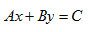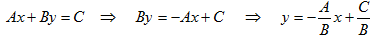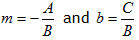Introduction

The slope-intercept form of the equation for a line states the slope of the equation explicitly, but the standard form does not. Since the slope is such an important feature of a line, it is useful to determine the slope if given the equation of the line in standard form. The standard form of the equation of a line is:Rewrite the equation in slope-intercept form by solving the equation for y.Compared with the slope-intercept form, y = mx + b, notice that. Remembering this relationship is useful, but solving the standard form for y will convert it to slope-intercept form where the slope and y -intercept are easily determined.

Listen